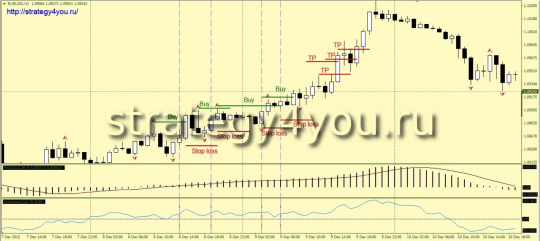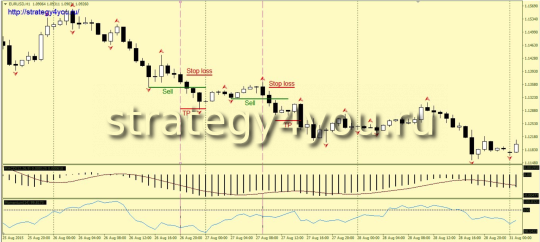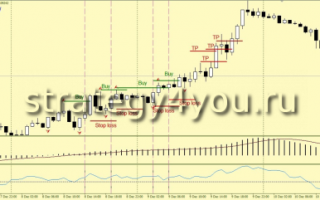# Strategy Forex «Moho»

Strategy Forex «Moho» is based on a set of standard indicators: MACD indicator defines the underlying trend (direction of trade), Momentum — shows the current mood of the market, and the Fractals indicator provides an entry point, so the strategy provides a good profit within a trend, however, it does not mean that is the trend would have to endure the drawdown. The lateral movements, this trading system continues to be profitable, but to a lesser pace.

Some statistics:

According to the currency pair EUR / USD from September 2015 until the end of December of the same year, the strategy showed the result + 1100 points (4-digit quotes). Maximum drawdown 150 points.

• Time interval — H1.
• Currency pair — any strategy multicurrency.

Indicators:

• Indicator Fractals
• MACD indicator with the parameters 12; 26; 9.
• Momentum indicator with period 14 and with an added level of 100.

## Conditions for entry on the BUY of forex «Moho» Strategy:1) The MACD indicator is above zero.

2) Momentum Indicator at the close of the last candle was above its level of 100.

3) above the nearest fractal upward, set the Buy Stop order to spread.

4) If the next candle closes so that the Momentum indicator is below its level of 100, this order will remove until the indicator again will not work above \$ 100.

5) Stop-loss is set at 30 points.

6) After a 23-25 ​​points in the positive zone trade should be transferred to breakeven.

7) Take-profit set at a distance of 60 points.

Attention: open existing transactions are not an obstacle to the opening of the following transactions with the new signal is received (another sample, the next fractal).

## Conditions for entrance for SELL on the «Moho» strategy:1) The MACD indicator is below its zero level.

2) Momentum indicator at the closing hour of the last candle is below the level of 100.

3) Following the nearest fractal, downward, set a pending order Sell Stop.

4) If the next candle closes so that the Momentum indicator will be higher than its level of 100, this order will remove until the indicator light will again be the 100.

5) The safety order is a stop-loss set at 30 points.

6) After the price of 23-25 ​​points in the positive zone, open trade should be transferred to the zero level.

7) The order of profit taking profit target is set at a distance of 60 points.

Having an open transaction are not an obstacle to opening additional transactions at the new signal is received in the same direction (the breakdown of another, the next fractal).